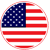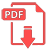# Home | Mathematics | Logarithms

## Logarithms

Logarithms are another way of thinking about exponents.
For example, we know that 2 raised to the 4th power equals 16. This is expressed by the exponential equation 24=16

Now, suppose someone asked us, "2 raised to which power equals 16?" The answer would be 4. This is expressed by the logarithmic equation log⁡2(16)=4 read as "log base two of sixteen is four".

24=16  ⟺  log⁡2(16)=4

Both equations describe the same relationship between the numbers 2, 4 and 16, where 2 is the base and 4 is the exponent.
The difference is that while the exponential form isolates the power 16 the logarithmic form isolates the exponent 4.
Here are more examples of equivalent logarithmic and exponential equations.

log⁡2(8)=3   ⟺   23=8
log⁡3(81)=4   ⟺   34=81
log⁡5(25)=2   ⟺   52=25English